## LCM and HCF

#### LCM and HCF

1. On being divided by a three digit number, the numbers 95336 and 91545 leave the same remainder. Find the number and the remainder.

1. Let the remainder in each case be t.
Then, the numbers (95336 – t) and (91545 – t) will be exactly divisible by that three digit number. As discussed earlier, the difference of the two numbers, i.e.,
[(95336 – t) – (91545 – t)] or, 3791 is exactly divisible by the three digit number. In other words, that three digit number will be a factor of 3791.
Now, 3791= 17 × 223
Since, the factors of 3791 have only 223 as three digit factor.

##### Correct Option: C

Let the remainder in each case be t.
Then, the numbers (95336 – t) and (91545 – t) will be exactly divisible by that three digit number. As discussed earlier, the difference of the two numbers, i.e.,
[(95336 – t) – (91545 – t)] or, 3791 is exactly divisible by the three digit number. In other words, that three digit number will be a factor of 3791.
Now, 3791= 17 × 223
Since, the factors of 3791 have only 223 as three digit factor.
So, Required number = 223
Now, we divide 95336 by 223 to get remainder.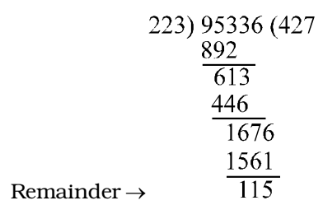1. The numbers 2270, 3739 and 6677 on being divided by a certain number of three digits, leave the same remainder. Find the number and the remainder respectively.

1. As done in the previous question,
The greatest common divisor = HCF of (3739 – 2270), (6677 – 3739) and (6677 – 2270)
= HCF of 1469, 2938 and 4407
Now, 1469 = 1469 × 1
2938 = 1469 × 2
4407 = 1469 × 3
∴ HCF = 1469
Now, 1469 = 113 × 13
Since, (2270 – R), (3739 – R) and (6677 – R), where R is the remainder, are exactly divisible by 1469, hence these are also exactly divisible by its factors 13 and 113. The three digit number is 113.

##### Correct Option: B

As done in the previous question,
The greatest common divisor = HCF of (3739 – 2270), (6677 – 3739) and (6677 – 2270)
= HCF of 1469, 2938 and 4407
Now, 1469 = 1469 × 1
2938 = 1469 × 2
4407 = 1469 × 3
∴ HCF = 1469
Now, 1469 = 113 × 13
Since, (2270 – R), (3739 – R) and (6677 – R), where R is the remainder, are exactly divisible by 1469, hence these are also exactly divisible by its factors 13 and 113. The three digit number is 113. Now the above mentioned numbers can be written as
2270 = (113 × 20 ) + 10
3739 = (113 × 33) + 10
6677 = (113 × 59) + 10
Hence, the required number is 113 and the remainder is 10.

1. On dividing the numbers 7654, 8506 and 9997 by a certain largest number, in each case the remainder is the same. Find the number and the remainder.

1. Let the remainder be t. Then (7654 – t), (8506 – t) and (9997 – t) are exactly divisible by that number.
Hence the required number
= HCF of (7654 – t),(8506 – t) and (9997 – t)
= HCF of (8506 – t) – (7654 – t), (9997 – t) – (8506 – t) and (9997 – t) – (7654 – t) = HCF of 852, 1491 and 2343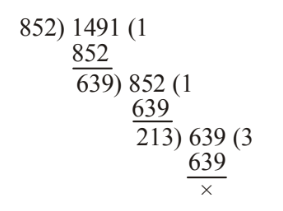Now, HCF of 213 and 2343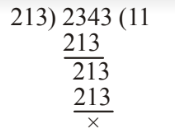Hence required number = 213
Required remainder = 7654 ÷ 213

##### Correct Option: A

Let the remainder be t. Then (7654 – t), (8506 – t) and (9997 – t) are exactly divisible by that number.
Hence the required number
= HCF of (7654 – t),(8506 – t) and (9997 – t)
= HCF of (8506 – t) – (7654 – t), (9997 – t) – (8506 – t) and (9997 – t) – (7654 – t) = HCF of 852, 1491 and 2343Now, HCF of 213 and 2343Hence required number = 213
Required remainder = 7654 ÷ 213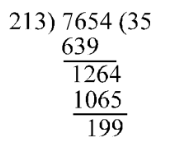Hence required remainder = 199

1. Find the greatest number which divides 99, 123 and 183 leaving the same remainder in each case.

1. Let t be the remainder. Then (99 – t), (123 – t) and (183 – t) will be exactly divisible by the required number. As discussed under division method of HCF, any number which divides the given number, also divides their difference. In other words, HCF of given numbers is same as the HCF of their difference.
∴ Required number = HCF of (123 – t) – (99 – t), (183 – t) – (123 – t) and (183 – t) – (99 – t)
Required number = HCF of (123 – 99), (183 – 123) and (183 – 99)
Required number = HCF of 24, 60 and 84
Now, 24 = 2 × 2 × 2 × 3
60 = 2 × 2 × 3 × 5
84 = 2 × 2 × 3 × 7

##### Correct Option: C

Let t be the remainder. Then (99 – t), (123 – t) and (183 – t) will be exactly divisible by the required number. As discussed under division method of HCF, any number which divides the given number, also divides their difference. In other words, HCF of given numbers is same as the HCF of their difference.
∴ Required number = HCF of (123 – t) – (99 – t), (183 – t) – (123 – t) and (183 – t) – (99 – t)
Required number = HCF of (123 – 99), (183 – 123) and (183 – 99)
Required number = HCF of 24, 60 and 84
Now, 24 = 2 × 2 × 2 × 3
60 = 2 × 2 × 3 × 5
84 = 2 × 2 × 3 × 7
∴ Required HCF = 2 × 2 × 3 = 12
∴ Required number = 13

1. Find the greatest number which will divide 42, 49 and 56 and leave remainders 6, 7 and 8 respectively.

1. Take the HCF of (42 – 6) = 36, (49 – 7) = 42 and (56 – 8) = 48
We find the HCF of 36 , 42 and 48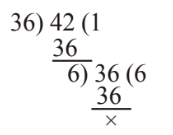##### Correct Option: A

Take the HCF of (42 – 6) = 36, (49 – 7) = 42 and (56 – 8) = 48
We find the HCF of 36 , 42 and 48And, HCF of 6 and 48 is also 6.
So, the required greatest number will be 6.